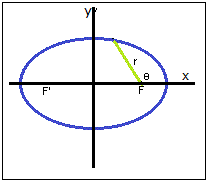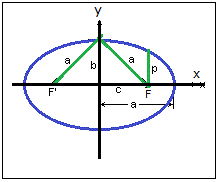# Axes of an ellipse

sutupidmath
in my textbook says that a^2=p^2/(1-e^2)^2, and b^2=p^2/(1-e^2), are two axes of an ellipse, however there is no any proof as to how we can be sure that a and b are such axes. Where p is the focal parameter, and e is the eccentricity of the ellipse; a- is the big semi-axes, b- the small one.So i would like to know is there any proof that ensures us that the above expressions are indeed or represent the axes of any ellipse??
I asked the assistant proffesor on my Analytical Geometry class, but she did not know how to proof it.

Any help would be welcomed.

Last edited:

## Answers and Replies

Gold MemberThe polar equation of an ellipse is ##r = \frac{p}{1 + e\cos \theta}##, where ##p## is the semi-latus rectum and ##e## is the eccentricity of the ellipse. The origin is a focus e.g. ##F## of the ellipse. The other focus of the ellipse is ##F'##. When ##\theta = 0^0## we have ##cos \theta = 1## and for ##\theta = 180^0##, ##\cos \theta = -1##. So, if ##a## is the semi-major axis, we have:
##2a = \frac{p}{1 + e} + \frac{p}{1 - e} = \frac{2p}{1 - e^2}##. Hence, ##a = \frac{p}{1 - e^2}##.Now, let ##c## be the distance from the center (coordinate axes intersection) to either focus, let's say ##F##.
We see that ##c = a - \frac{p}{1 + e} = \frac{ep}{1 - e^2} = ea##. If ##b## is the semi-minor axis - e.g. in the upper half of the ellipse in the above diagram, and if we draw a line that connects the focus ##F## to the point that semi-minor axis ##b## intersects the ellipse, we have a right triangle. Its two perpendicular sides are ##c## and ##b## and its hypotenuse is ##a##. Using Pythagorean theorem and utilizing the fact that ##c = ea##, as we saw above, we find that ##b = a \sqrt{1 - e^2}##. Now, using the expression we obtained before for ##a## we have

##b = \frac{p}{1 - e^2} \cdot \sqrt{1 - e^2}##

So, the (semi)axes ##a## and ##b## can indeed be written in a way, such that, if we square the final expressions we found, we have the expressions asked in the OP.